International
Tables for
Crystallography
Volume C
Mathematical, physical and chemical tables
Edited by E. Prince

International Tables for Crystallography (2006). Vol. C, ch. 8.1, pp. 685-687

## Section 8.1.5. Numerical methods for large-scale problems

E. Princea and P. T. Boggsb

aNIST Center for Neutron Research, National Institute of Standards and Technology, Gaithersburg, MD 20899, USA, and bScientific Computing Division, National Institute of Standards and Technology, Gaithersburg, MD 20899, USA

### 8.1.5. Numerical methods for large-scale problems

| top | pdf |

Because the least-squares problems arising in crystallography are often very large, the methods we have discussed above are not always the most efficient. Some large problems have special structure that can be exploited to produce quite efficient algorithms. A particular special structure is sparsity, that is, the problems have Jacobian matrices that have a large fraction of their entries zero. Of course, not all large problems are sparse, so we shall also discuss approaches applicable to more general problems.

#### 8.1.5.1. Methods for sparse matrices

| top | pdf |

We shall first discuss large, sparse, linear least-squares problems (Heath, 1984), since these techniques form the basis for nonlinear extensions. As we proceed, we shall indicate how the procedures should be modified in order to handle nonlinear problems. Recall that the problem is to find the minimum of the quadratic form [yAx]TW[yAx], where y is a vector of observations, Ax represents a vector of the values of a set of linear model functions that predict the values of y, and W is a positive-definite weight matrix. Again, for convenience, we make the transformation y′ = Uy, where U is the upper triangular Cholesky factor of W, and Z = UA, so that the quadratic form becomes (y′ − Zx)T(y′ − Zx), and the minimum is the solution of the system of normal equations, ZTZx = ZTy′. Even if Z is sparse, it is easy to see that H = ZTZ need not be sparse, because if even one row of Z has all of its elements nonzero, all elements of H will be nonzero. Therefore, the direct use of the normal equations may preclude the efficient exploitation of sparsity. But suppose H is sparse. The next step in solving the normal equations is to compute the Cholesky decomposition of H, and it may turn out that the Cholesky factor is not sparse. For example, if H has the form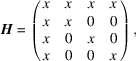where x represents a nonzero element, then the Cholesky factor, R, will not be sparse, but if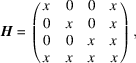then R has the form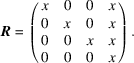These examples show that, although the sparsity of R is independent of the row ordering of Z, the column order can have a profound effect. Procedures exist that analyse Z and select a permutation of the columns that reduces the `fill' in R. An algorithm for using the normal equations is then as follows:

 (1) determine a permutation, P (an orthogonal matrix with one and only one 1 in each row and column, and all other elements zero), that tends to ensure a sparse Cholesky factor; (2) store the elements of R in a sparse format; (3) compute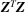and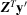; (4) factor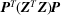to get R; (5) solve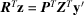; (6) solve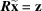; (7) set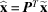.

This algorithm is fast, and it will produce acceptable accuracy if the condition number of Z is not too large. If extension to the nonlinear case is considered, it should be kept in mind that the first two steps need only be done once, since the sparsity pattern of the Jacobian does not, in general, change from iteration to iteration.

The QR decomposition of matrices that may be kept in memory is most often performed by the use of Householder transformations (see Subsection 8.1.1.1). For sparse matrices, or for matrices that are too large to be held in memory, this technique has several drawbacks. First, the method works by inserting zeros in the columns of Z, working from left to right, but at each step it tends to fill in the columns to the right of the one currently being worked on, so that columns that are initially sparse cease to be so. Second, each Householder transformation needs to be applied to all of the remaining columns, so that the entire matrix must be retained in memory to make efficient use of this procedure.

The alternative procedure for obtaining the QR decomposition by the use of Givens rotations overcomes these problems if the entire upper triangular matrix, R, can be stored in memory. Since this only requires about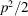locations, it is usually possible. Also, it may happen that R has a sparse representation, so that even fewer locations will be needed. The algorithm based on Givens rotations is as follows:

• (1) bring in the first p rows;

• (2) find the QR decomposition of this p × p matrix;

• (3) for i = p + 1 to n, do

 (a) bring in row i; (b) eliminate row i using R and at most p Givens rotations.

In order to specify how to use this algorithm to solve the linear least-squares problem, we must also state how to account for Q. We could accumulate Q or save enough information to generate it later, but this usually requires excessive storage. The better alternatives are either to apply the steps of Q to y′ as we proceed or to simply discard the information and solve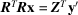. It should be noted that the order of rows can make a significant difference. Suppose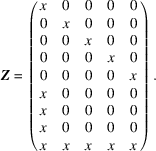The work to complete the QR decomposition is of order p2 operations, because each element below the main diagonal can be eliminated by one Givens rotation with no fill, whereas for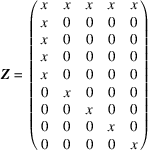each Givens rotation fills an entire row, and the QR decomposition requires of order np2operations.

#### 8.1.5.2. Conjugate-gradient methods

| top | pdf |

A numerical procedure that is applicable to large-scale problems that may not be sparse is called the conjugate-gradient method. Conjugate-gradient methods were originally designed to solve the quadratic minimization problem, find the minimum of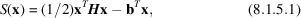where H is a symmetric, positive-definite matrix. The gradient of S is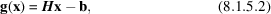and its Hessian matrix is H. Given an initial estimate,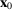, the conjugate-gradient algorithm is

• (1) define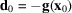;

• (2) for k = 0, 1, 2, ..., p − 1,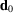(a)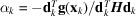; (b)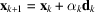; (c)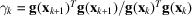; (d)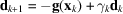.

This algorithm finds the exact solution for the quadratic function in not more than p steps.

This algorithm cannot be used directly for the nonlinear case because it requires H to compute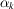, and the goal is to solve the problem without computing the Hessian. To accomplish this, the exact computation of α is replaced by an actual line search, and the termination after at most p steps is replaced by a convergence test. Thus, we obtain, for a given starting valueand a general, nonquadratic function S:

• (1) define;

• (2) set k = 0;

• (3) do until convergence

 (a), whereis chosen by a line search; (b); (c)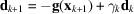; (d)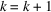.

Note that, as promised, H is not needed. In practice, it has been observed that the line search need not be exact, but that periodic restarts in the steepest-descent direction are often helpful. This procedure often requires more iterations and function evaluations than methods that store approximate Hessians, but the cost per iteration is small. Thus, it is often the overall least-expensive method for large problems.

For the least-squares problem, recall that we are finding the minimum of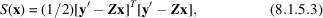for which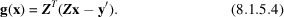By using these definitions in the conjugate-gradient algorithm, it is possible to formulate a specific algorithm for linear least squares that requires only the calculation of Z times a vector and ZT times a vector, and never requires the calculation or factorization of ZTZ.

In practice, such an algorithm will, due to roundoff error, sometimes require more than p iterations to reach a solution. A detailed examination of the performance of the procedure shows, however, that fewer than p iterations will be required if the eigenvalues of ZTZ are bunched, that is, if there are sets of multiple eigenvalues. Specifically, if the eigenvalues are bunched into k distinct sets, then the conjugate-gradient method will converge in k iterations. Thus, significant improvements can be made if the problem can be transformed to one with bunched eigenvalues. Such a transformation leads to the so-called preconditioned conjugate-gradient method. In order to analyse the situation, let C be a p × p matrix that transforms the variables, such that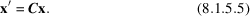Then,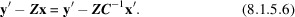Therefore, C should be such that the system Cx = x′ is easy to solve, and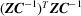has bunched eigenvalues. The ideal choice would be C = R, where R is the upper triangular factor of the QR decomposition, since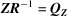.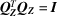has all of its eigenvalues equal to one, and, since R is triangular, the system is easy to solve. If R were known, however, the problem would already be exactly solved, so this is not a useful alternative. Unfortunately, no universal best choice seems to exist, but one approach is to choose a sparse approximation to R by ignoring rows that cause too much fill in or by making R a diagonal matrix whose elements are the Euclidean norms of the columns of Z. Bear in mind that, in the nonlinear case, an expensive computation to choose C in the first iteration may work very well in subsequent iterations with no further expense. One should be aware of the trade off between the extra work per iteration of the preconditioned-conjugate gradient method versus the reduction in the number of iterations. This is especially important in nonlinear problems.

The solution of large, least-squares problems is currently an active area of research, and we have certainly not given an exhaustive list of methods in this chapter. The choice of method or approach for any particular problem is dependent on many conditions. Some of these are:

 (1) The size of the problem. Clearly, as computer memories continue to grow, the boundary between small and large problems also grows. Nevertheless, even if a problem can fit into memory, its sparsity structure may be exploited in order to obtain a more efficient algorithm. (2) The number of times the problem (or similar ones) will be solved. If it is a one-shot problem (a rare occurrence), then one is usually most strongly influenced by easy-to-use, existing software. Exceptions, of course, exist where even a single solution of the problem requires extreme care. (3) The expense of evaluating the function. With a complicated, nonlinear function like the structure-factor formula, the computational effort to determine the values of the function and its derivatives usually greatly exceeds that required to solve the linearized problem. Therefore, a full Gauss–Newton, trust-region, or quasi-Newton method may be warranted. (4) Other structure in the problem. Rarely does a problem have a random sparsity pattern. Non-zero values usually occur in blocks or in some regular pattern for which special decomposition methods can be devised. (5) The machine on which the problem is to be solved. We have said nothing about the existing vector and parallel processors. Suffice it to say that the most efficient procedure for a serial machine may not be the right algorithm for one of these novel machines. Appropriate numerical methods for such architectures are also being actively investigated.

### References

Heath, M. T. (1984). Numerical methods for large, sparse, linear least squares problems. SIAM J. Sci. Stat. Comput. 5, 497–513.